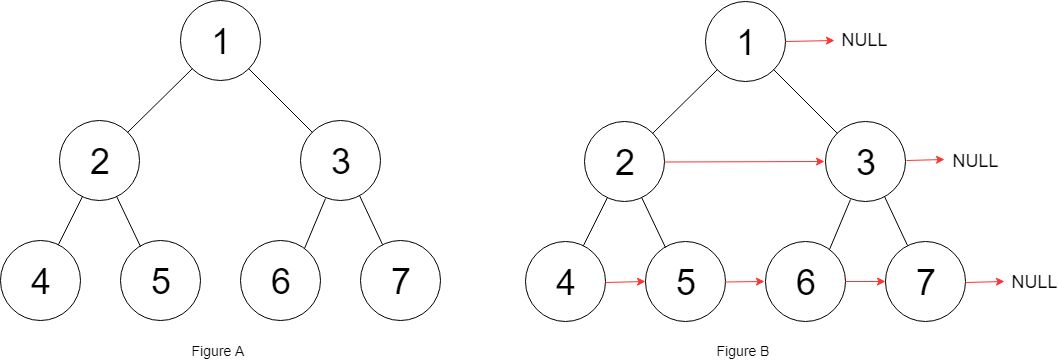## Algorithm

Problem Nmae: 116. Populating Next Right Pointers in Each Node

You are given a perfect binary tree where all leaves are on the same level, and every parent has two children. The binary tree has the following definition:

```struct Node {
int val;
Node *left;
Node *right;
Node *next;
}
```

Populate each next pointer to point to its next right node. If there is no next right node, the next pointer should be set to `NULL`.

Initially, all next pointers are set to `NULL`.

Example 1:```Input: root = [1,2,3,4,5,6,7]
Output: [1,#,2,3,#,4,5,6,7,#]
Explanation: Given the above perfect binary tree (Figure A), your function should populate each next pointer to point to its next right node, just like in Figure B. The serialized output is in level order as connected by the next pointers, with '#' signifying the end of each level.
```

Example 2:

```Input: root = []
Output: []
```

Constraints:

• The number of nodes in the tree is in the range `[0, 212 - 1]`.
• `-1000 <= Node.val <= 1000`

## Code Examples

### #1 Code Example with C Programming

```Code - C Programming```

``````
if (!root || !root->left) return;
v = root;
while (v->left) {
h = v;
do {
h->left->next = h->right;
if (h->next) {
h->right->next = h->next->left;
}
h = h->next;
} while (h);
v = v->left;
}
}
``````
Copy The Code &

Input

cmd
root = [1,2,3,4,5,6,7]

Output

cmd
[1,#,2,3,#,4,5,6,7,#]

### #2 Code Example with C++ Programming

```Code - C++ Programming```

``````
class Solution {
public:
if(!root) return;
dequecur;
dequenext;
cur.push_back(root);
while(!cur.empty()){
cur.pop_front();
node->next = cur.empty() ? NULL : cur.front();
if(node->left) next.push_back(node->left);
if(node->right) next.push_back(node->right);
if(cur.empty()) swap(cur, next);
}
}
};
``````
Copy The Code &

Input

cmd
root = [1,2,3,4,5,6,7]

Output

cmd
[1,#,2,3,#,4,5,6,7,#]

### #3 Code Example with Java Programming

```Code - Java Programming```

``````
class Solution {
public Node connect(Node root) {
Node prev = root;
Node curr = null;
while (prev != null && prev.left != null) {
curr = prev;
while (curr != null) {
curr.left.next = curr.right;
if (curr.next != null) {
curr.right.next = curr.next.left;
}
curr = curr.next;
}
prev = prev.left;
}
return root;
}
}
``````
Copy The Code &

Input

cmd
root = []

Output

cmd
[]

### #4 Code Example with Javascript Programming

```Code - Javascript Programming```

``````
const connect = function(root) {
if (root == null) return null
const cur = [root]
while (cur.length) {
let len = cur.length
for (let i = 0; i < len; i++) {
let el = cur.shift()
if (i === len - 1) el.next = null
else el.next = cur

if (el.left) cur.push(el.left)
if (el.right) cur.push(el.right)
}
}
return root
}
``````
Copy The Code &

Input

cmd
root = []

Output

cmd
[]

### #5 Code Example with Python Programming

```Code - Python Programming```

``````
class Solution:
def connect(self, root: "Node") -> "Node":
if root == None:
return root
q, prev = [(root, 1)], None
while q:
curr, pos = q.pop(0)
if prev != None and prev == pos:
prev.next = curr
prev = [curr, pos]
if curr.left:
q.append((curr.left, pos + 1))
if curr.right:
q.append((curr.right, pos + 1))
return root
``````
Copy The Code &

Input

cmd
root = [1,2,3,4,5,6,7]

Output

cmd
[1,#,2,3,#,4,5,6,7,#]

### #6 Code Example with C# Programming

```Code - C# Programming```

``````
namespace LeetCode
{
public class _0116_PopulatingNextRightPointersInEachNode
{
{
while (levelStart != null)
{
while (cur != null)
{
if (cur.left != null) cur.left.next = cur.right;
if (cur.right != null && cur.next != null) cur.right.next = cur.next.left;

cur = cur.next;
}
levelStart = levelStart.left;
}

return root;
}
}
}
``````
Copy The Code &

Input

cmd
root = [1,2,3,4,5,6,7]

Output

cmd
[1,#,2,3,#,4,5,6,7,#]